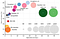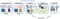# Loading and Training a Neural Network with Custom dataset via Transfer Learning in Pytorch

In the previous post (here), we loaded and transformed custom images from a directory of training and validation datasets into appropriately processed Tensors; now we are ready to load, modify, train and test an existing model with our readymade data, in four steps:

• Loading a Neural Network model
• Building the classifier and training the network
• Testing the modified network
• Saving the checkpoint

There are a variety of existing Neural Networks(NN), trained on vast amounts of datasets such as Imagenet, Kaggle and the UCI repository just to state a few. The graph below describes such public NN models, on a scale of the accuracy achieved upon conception, with respect to the dataset size used for training.top-1 one-crop accuracy over the number of operations required for a single forward pass in multiple popular neural network architectures

To load the NN model of a preferred type, import the ‘models’ package from ‘torchvision’ and call your desired model with the required parameters:

`#import models from torchvisionfrom torchvision import models#build the pretrained model (vgg16 in this case)model = models.vgg16(pretrained = True)`

NB: Out of the vast number of models, ‘vgg-16' is chosen. The pre-trained parameter is set to True because we want to start building from a pre-trained model with optimized weights and biases.

Like every other model architecture, vgg-16 is made up of a large number of convolution and pooling layers to extract spatial features, with fully connected layers at the end, consisting of the classifier. Here is where the most technical part — known as transfer Learning — comes into play.

Transfer learning is applied here, by modifying the classifier of the loaded NN with a new classifier, adapted to our datasets structure, mainly in terms of the dataset’s input feature size and expected output size. The following code snippet creates a classifier for our custom dataset, and is then added to the loaded vgg-16 model.

`#import OrderedDicted to corectly align the network layers#import nn you use activation and dropout featuresfrom collections import OrderedDictfrom torch import nn#create classifierclassifier         =nn.Sequential(OrderedDict([('fc1', nn.Linear(25088, 512)),                           ('relu', nn.ReLU()),                            ('dropout', nn.Dropout(p=0.337)),                           ('fc2', nn.Linear(512, 102)),                           ('output', nn.LogSoftmax(dim=1))                             ]))#replace the model's classifier with this new classifier #transfer learning connection applied heremodel.classifier = classifier`

NB: Two things worth noting here: The input size (25088 in this case) should be equivalent to that specified by the network, and the output size (102) should be equivalent to the number of all the classes represented by the dataset.

Now we train the network. This is done by first defining the loss function (Cross Entropy Loss is generally used) and the network optimizer (Stochastic Gradient Descent [SGD] in this case) with respective parameters:

`#import optimizer for from torch import optim#define criteria and optimizercriteria = nn.CrossEntropyLoss()optimizer = optim.SGD(model.parameters(), lr = 0.005, momentum = 0.5)`

The above code snippet sets the learning rate to 0.005 (the step size taken by a model to minimize loss and update weights with the goal to improve predictive accuracy) and momentum of 0.5 (the amount by which a model can easily bump off any local minima during the gradient descent process while searching for the global minimum).

The network can now be trained, one sample at a time, throughout the whole sample size; the process is repeated this time with updated weights, yielding better accuracies. The latter is done iteratively for a defined number of times. This process is cheaply and accurately achieved with two defined functions and an iterative loop from zero to the number of epochs times as follows:

• The first is the training function, which takes in the defined model, the dataset (training Loader) and the loss criterion, then returns the loss and accuracy achieved for each epoch as follows:
`#define training functiondef train (model, loader, criterion, gpu):    model.train()    current_loss = 0    current_correct = 0    for train, y_train in iter(loader):        if gpu:            train, y_train = train.to('cuda'), y_train.to('cuda')        optimizer.zero_grad()        output = model.forward(train)        _, preds = torch.max(output,1)        loss = criterion(output, y_train)        loss.backward()        optimizer.step()        current_loss += loss.item()*train.size(0)        current_correct += torch.sum(preds == y_train.data)    epoch_loss = current_loss / len(trainLoader.dataset)    epoch_acc = current_correct.double() / len(trainLoader.dataset)            return epoch_loss, epoch_acc`
• The second is the validation function, which takes in the defined model, the dataset (validation Loader) and the loss criterion; it also returns the loss and accuracy for each epoch as follows:
`#define validation functiondef validation (model, loader, criterion, gpu):    model.eval()    valid_loss = 0    valid_correct = 0    for valid, y_valid in iter(loader):        if gpu:            valid, y_valid = valid.to('cuda'), y_valid.to('cuda')        output = model.forward(valid)        valid_loss += criterion(output, y_valid).item()*valid.size(0)        equal = (output.max(dim=1) == y_valid.data)        valid_correct += torch.sum(equal)#type(torch.FloatTensor)        epoch_loss = valid_loss / len(validLoader.dataset)    epoch_acc = valid_correct.double() / len(validLoader.dataset)        return epoch_loss, epoch_acc`

NB: Two things worth noting here. The training function switches the model to training mode and initializes the optimizer gradients to zero, before updating. On the other hand, the validation function instead switches the model to evaluation mode and receives the updated gradients from the training function, for evaluation.

The last step here is to combine both functions in a loop for the number of epochs times (20 in this case). During each iteration, the loss and accuracy produced by each function are displayed. Following is the code snippet:

`#Initialize training params  #freeze gradient parameters in pretrained modelfor param in model.parameters():    param.require_grad = False#train and validateepochs = 10  epoch = 0#send model to GPUif args.gpu:    model.to('cuda')    for e in range(epochs):    epoch +=1    print(epoch)    with torch.set_grad_enabled(True):        epoch_train_loss, epoch_train_acc = train(model,trainLoader, criteria, args.gpu)    print("Epoch: {} Train Loss : {:.4f}  Train Accuracy: {:.4f}".format(epoch,epoch_train_loss,epoch_train_acc))with torch.no_grad():        epoch_val_loss, epoch_val_acc = validation(model, validLoader, criteria, args.gpu)    print("Epoch: {} Validation Loss : {:.4f}  Validation Accuracy {:.4f}".format(epoch,epoch_val_loss,epoch_val_acc))`

NB: Two things worth noting here: Gradients must be turned true during the training phase, then turned off during the validation phase before calling the respective functions. Secondly, the validation loss should generally be lower compared to the training loss at the current epoch (iteration), and at the same time, the validation accuracy should be higher compared to the training accuracy. Combined with the latter observation, if the loss is constantly decreasing while the accuracy constantly increases, then you are in the light of producing an accurate network for your custom dataset.

Having trained and evaluated our Network with a good accuracy, we are more than ready to test the network with new data of related classes.

It is good practice to always test the trained network on test data which the network has never seen either in training nor validation. This gives a good estimate for the model’s performance on completely new inputs. Here, we pass the test images through the network and measure the accuracy just as in the validation function:

`model.eval()total = 0correct = 0 count = 0#iterating for each sample in the test dataset oncefor test, y_test in iter(testLoader):    test, y_test = test.to('cuda'), y_test.to('cuda')#Calculate the class probabilities (softmax) for img    with torch.no_grad():        output = model.forward(test)        ps = torch.exp(output)        _, predicted = torch.max(output.data,1)        total += y_test.size(0)        correct += (predicted == y_test).sum().item()         count += 1        print("Accuracy of network on test images is ... {:.4f}....count: {}".format(100*correct/total,  count ))`

The above code snippet tests the input images with no class labels and predicts which image belongs to which class, with an associated percentage of confidence.

From a general standpoint, the following visual depicts what the algorithm does thus far:Transfer Learning process

Because we want to avoid time and computation wastage, it is best practice to always save a model’s checkpoint. It should be a relief noting that the ‘vgg-16’ model we loaded was a checkpoint saved by some other person in the first place, and we used the resulting features and weights of the model, without having to train everything from scratch. We also want to save our model, in order to enable a third party to use it, especially if the datasets are similar. Torch provides a save function to save our models. All that is required as parameters are the model and the directory path where we want the checkpoint to be saved:

`#create the checkpoint and save every sensitive information starting #from the model state dictionary, model criterion, optimizer, to the #number of epochscheckpoint = {'model_state': model.state_dict(),              'criterion_state': criteria.state_dict(),              'optimizer_state': optimizer.state_dict(),              'class_to_idx': train_datasets.class_to_idx,              'epochs': epochs,              'Best train loss': epoch_train_loss,              'Best train accuracy': epoch_train_accuracy,              'Best Validation loss': epoch_val_loss,              'Best Validation accuracy': epoch_val_acc}torch.save(checkpoint, args.checkpoint)`

We have a custom dataset of training validation and testing (from the previous post here); we just creating a model adapted to our dataset via transfer learning; we trained and validated the model while tuning a number of hyperparameters such as learning rate, epoch size, batch size, momentum; we tested the performance of our model with test data, by predicting the classes of each test sample with competitive accuracies; we saved our model as a checkpoint in a given directory. Now we can confidently deploy our model on any platform, and let it do some predictive work for us.

In all, by modifying our dataset to that of an appropriate situation at hand (consisting mostly of a cleaning and analysis phase), then building on an existing model or creating a new model, we are able to solve a vast number of problems ranging from image recognition to quantitative analysis (stock price prediction), credit risk modeling, insurance risk modeling, credit card fraud detection, twitter messaging analysis and much more.

In the next post, we are going to work with dockers and create related apps in the enterprise environment.

#DataIsPower

Good Luck!